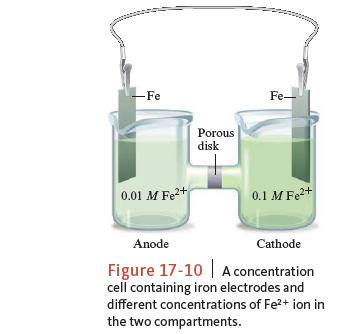# Problem: Consider the concentration cell in Fig. 17‑10. If the Fe 2+ concentration in the right compartment is changed from 0.1 M to 1 x 10-7 M Fe2+, predict the direction of electron flow, and designate the anode and cathode compartments.

###### FREE Expert Solution

electrons flow from the anode to the cathode

anode decrease concentration

cathode increase concentration

82% (217 ratings)###### Problem Details

Consider the concentration cell in Fig. 17‑10. If the Fe 2+ concentration in the right compartment is changed from 0.1 M to 1 x 10-7 M Fe2+, predict the direction of electron flow, and designate the anode and cathode compartments.Frequently Asked Questions

What scientific concept do you need to know in order to solve this problem?

Our tutors have indicated that to solve this problem you will need to apply the The Nernst Equation concept. You can view video lessons to learn The Nernst Equation. Or if you need more The Nernst Equation practice, you can also practice The Nernst Equation practice problems.

What textbook is this problem found in?

Our data indicates that this problem or a close variation was asked in Chemistry: An Atoms First Approach - Zumdahl Atoms 1st 2nd Edition. You can also practice Chemistry: An Atoms First Approach - Zumdahl Atoms 1st 2nd Edition practice problems.